## Overview🔗

Flow physics:

• External flow
• High Reynolds number
• Low Mach number, subsonic
• Newtonian, single-phase, incompressible, non-reacting

Solver:

Tutorial case:

## Physics and Numerics🔗

Physical domain:

• The case is a two-dimensional airfoil located around the centre of a computational domain whose dimensions are considerably larger than the chord-length of the airfoil.
• $$x$$: Longitudinal direction (mean flow direction)
• $$y$$: Spanwise direction (statistically homogeneous direction)
• $$z$$: Vertical direction (wall-normal direction)
• $$O$$: Origin at the leading edge of the airfoil

Physical modelling:

• Reynolds number based on local chord length: $$\text{Re}_c = U_x \, c \, \nu^{-1} \approx 6\times10^6$$
• Streamwise far-field flow speed: $$U_x = 51.4815$$ [m⋅s-1]
• Characteristic length (Local chord length of the airfoil): $$c = 1.0$$ [m]
• Kinematic viscosity of fluid: $$\nu_\text{fluid} = 8.58 \times 10^{-6}$$ [m2⋅s-1]
• Mach number: $$\text{Ma} = U_x / U_s \approx 0.15$$
• Speed of sound: $$U_s = 343.21$$ [m⋅s-1]
• Turbulence model: Spalart Allmaras

Numerical domain modelling:

• Shape: extruded C-grid
• Dimensions: $$(x, y, z) \approx (985.5, 1.0, 1015.6)$$ [m]
• Sketch (View direction to $$y$$-positive):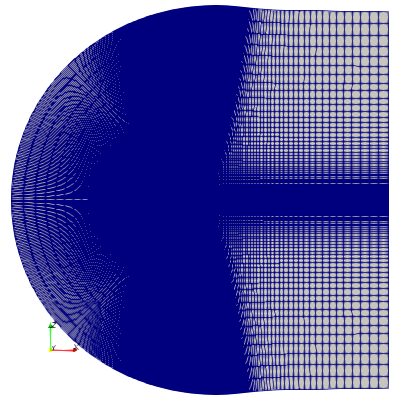Spatial domain discretisation:

• Mesh type: hexahedral cells in plot3d format
• Mesh converter: plot3dToFoam
• Number of cells, $$N$$ : $$(N_x, N_y, N_z) = (257, 1, 897)$$
• First wall-normal cell centre height: $$\Delta_y^+ < 1$$
• Mesh detail (View direction to $$y$$-positive):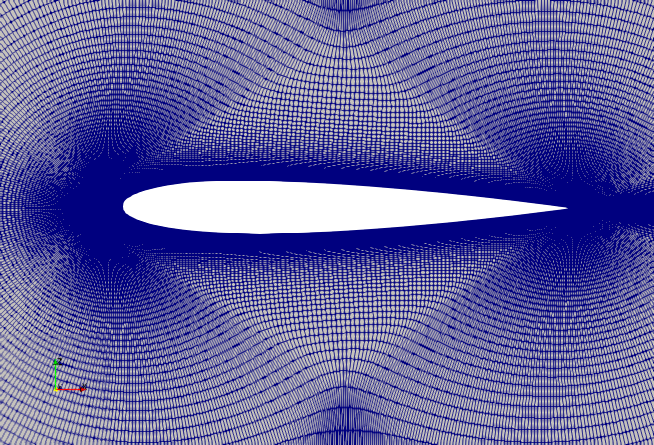Equation discretisation:

Spatial derivatives and variables:

• Divergence:
• default: Gauss linear
• div(phi,U): bounded Gauss linearUpwind grad(U)
• div(phi,nuTilda): bounded Gauss linearUpwind grad(nuTilda)
• Laplacian: Gaussian linear corrected

Temporal derivatives and variables:

Numerical boundary conditions:

• Velocity, $$\mathbf{U}$$
Patch Condition Value [m⋅s-1]
Inlet freestreamVelocity $$\mathbf{U}_\alpha$$
Outlet freestreamVelocity $$\mathbf{U}_\alpha$$
Sides $$\text{(}y$$-dir) empty -
Aerofoil fixedValue (0.0, 0.0, 0.0)
α U α
$$\alpha = 0^o$$ (51.4815, 0.00, 0.0000)
$$\alpha = 10^o$$ (50.6994, 0.00, 8.9397)
$$\alpha = 15^o$$ (49.7273, 0.00, 13.3244)
• Kinematic pressure, p
Patch Condition Value [m2⋅s-2]
Inlet freestreamPressure 0.0
Outlet freestreamPressure 0.0
Sides $$\text{(}y$$-dir) empty -
• Turbulent kinematic viscosity, nut (i.e. $$\nu_t$$)
Patch Condition Value [m2⋅s-1]
Inlet freestream $$8.58e^{-6} \approx \nu_\text{fluid}$$ 
Outlet freestream $$8.58e^{-6}\approx \nu_\text{fluid}$$ 
Sides $$\text{(}y$$-dir) empty -
Aerofoil fixedValue 0.0 
• Spalart-Allmaras model modified viscosity, nuTilda (i.e. $$\tilde{\nu}$$)
Patch Condition Value [m2⋅s-1]
Inlet freestream $$3.432e^{-5} \approx 4 \nu_\text{fluid}$$ 
Outlet freestream $$3.432e^{-5}\approx 4 \nu_\text{fluid}$$ 
Sides $$\text{(}y$$-dir) empty -
Aerofoil fixedValue 0.0 

Solution algorithms and solvers:

• Pressure-velocity: SIMPLE
• Parallel decomposition of spatial domain and fields: Not applicable
• Linear solvers:
Field Linear Solver Smoother Tolerance (rel)
U smooth GaussSeidel 0.01
p GAMG GaussSeidel 0.01
nuTilda smooth GaussSeidel 0.01

## Results🔗

List of metrics:

• Lift coefficient $$\mathrm{C}_\mathrm{L}$$ vs. Angle of attack $$\alpha$$
• Drag coefficient $$\mathrm{C}_\mathrm{D}$$ vs. Angle of attack $$\alpha$$
• Drag coefficient $$\mathrm{C}_\mathrm{D}$$ vs. Lift coefficient $$\mathrm{C}_\mathrm{L}$$
• Surface pressure coefficient $$\mathrm{C}_p$$ vs. Normalised chord length $$x/c$$
• Surface skin friction coefficient $$\mathrm{C}_f$$ vs. Normalised chord length $$x/c$$
• $$\{\overline{\cdot}\}$$ is the time-averaging operator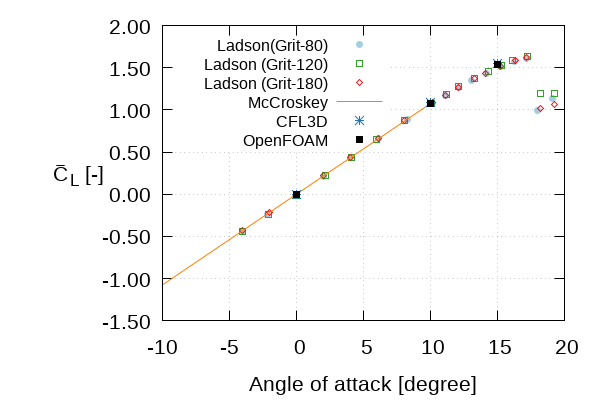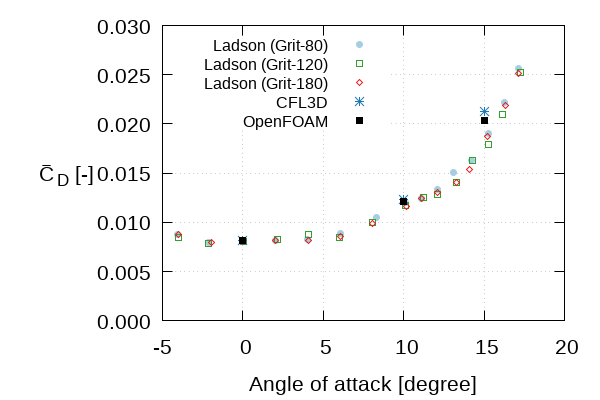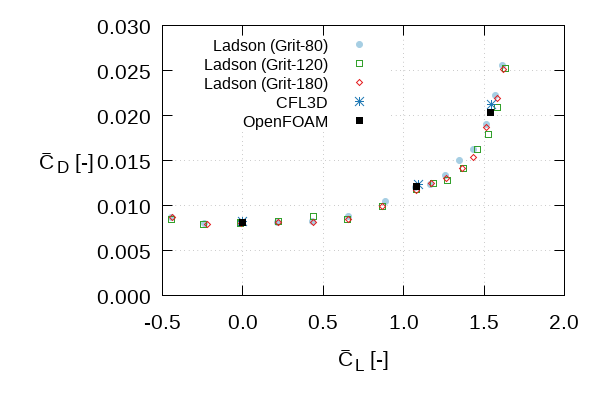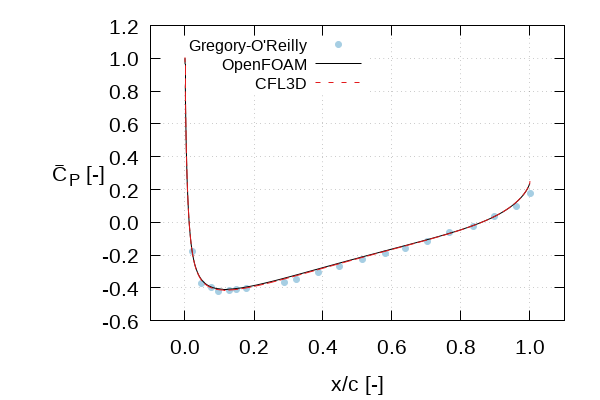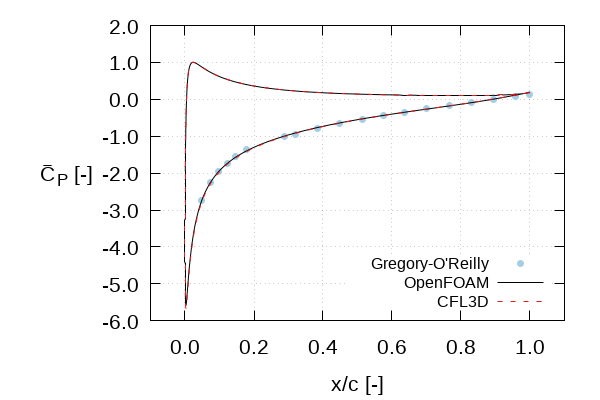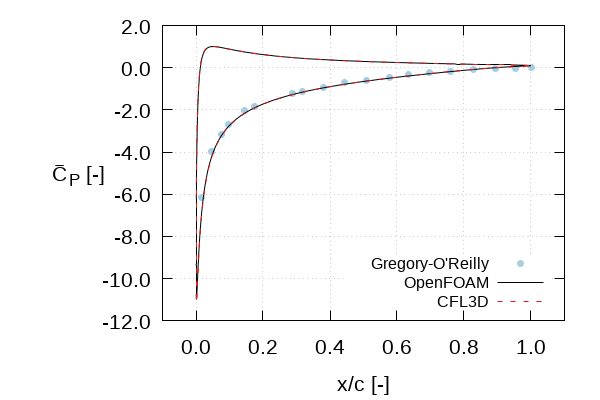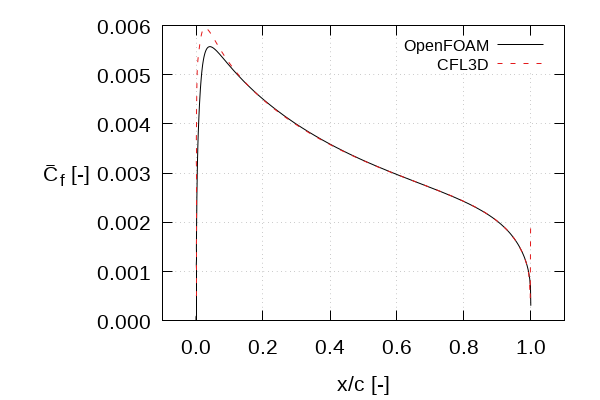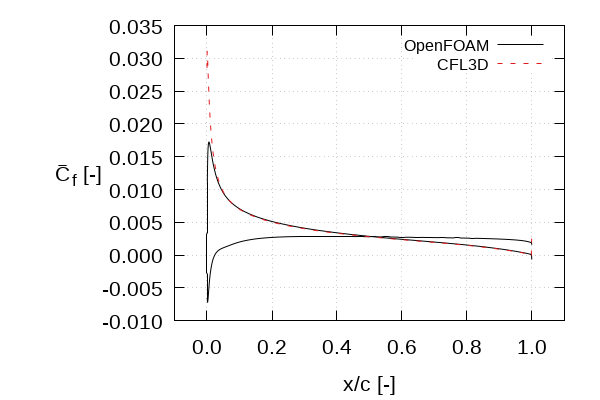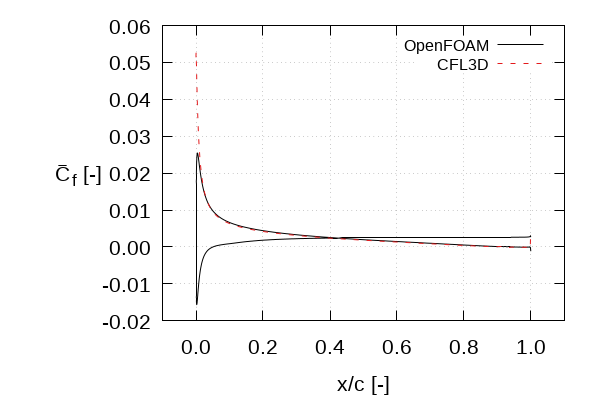## Resources🔗

Note: Links will take you to the NASA website

### Datasets for verifications (plain text)🔗

Lift and drag coefficients vs angle of attack

Pressure distribution vs local chord length

Lift coefficient vs angle of attack

Skin friction coefficient vs local chord length